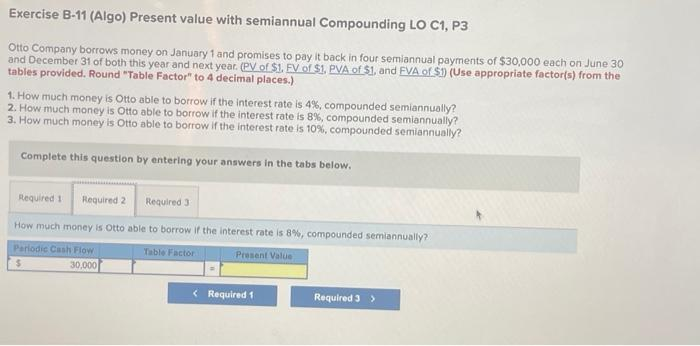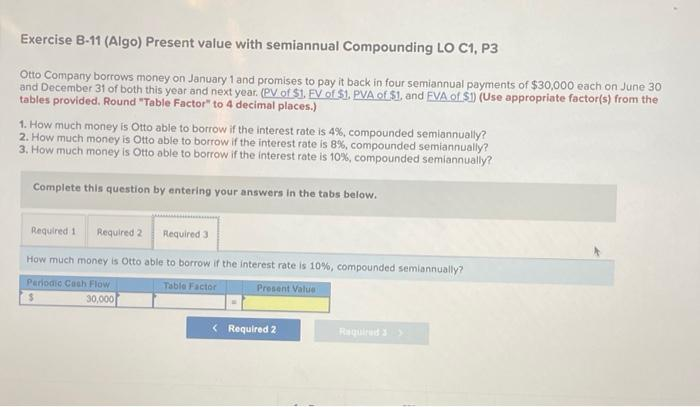Home / Expert Answers / Accounting / this-is-all-1-question-theres-just-three-parts-to-it-exercise-b-11-algo-present-value-with-semia-pa672

# (Solved): this is all 1 question, theres just three parts to it Exercise B-11 (Algo) Present value with semia ...

this is all 1 question, theres just three parts to itExercise B-11 (Algo) Present value with semiannual Compounding LO C1, P3 Otto Company borrows money on January 1 and promises to pay it back in four semiannual payments of each on June 30 and December 31 of both this year and next year. (PV of \$1, FV of \$1. PVA of \$1, and EVA of \$1) (Use appropriate factor(s) from the tables provided. Round "Table Factor" to 4 decimal places.) 1. How much money is Otto able to borrow if the interest rate is , compounded semiannually? 2. How much money is Otto able to borrow if the interest rate is , compounded semiannually? 3. How much money is Otto able to borrow if the interest rate is , compounded semiannually? Complete this question by entering your answers in the tabs below. How much money is Otto able to borrow if the interest rate is , compounded semiannuaily? Exercise B-11 (Algo) Present value with semiannual Compounding LO C1, P3 Otto Company borrows money on January 1 and promises to pay it back in four semiannual payments of each on June 30 and December 31 of both this year and next year. (PV of \$1, FV of \$1, PVA of \$1, and FVA of \$1) (Use appropriate factor(s) from the tables provided, Round "Table Factor" to 4 decimal places.) 1. How much money is Oto able to borrow if the interest rate is , compounded semiannually? 2. How much money is Otto able to borrow if the interest rate is , compounded semiannually? 3. How much money is Otto able to borrow if the interest rate is , compounded semiannually? Complete this question by entering your answers in the tabs below. How much money is otto able to borrow if the interest rate is , compounded semiannually? Exercise B-11 (Algo) Present value with semiannual Compounding LO C1, P3 Otto Company borrows money on January 1 and promises to pay it back in four semiannual payments of each on June 30 and December 31 of both this year and next year. (PV of \$1, FV of \$1, PVA of \$1, and EVA of \$1) (Use appropriate factor(s) from the tables provided, Round "Table Factor" to 4 decimal places.) 1. How much money is Otto able to borrow if the interest rate is , compounded semiannually? 2. How much money is Otto able to borrow if the interest rate is , compounded semiannually? 3. How much money is Otto able to borrow if the interest rate is , compounded semiannually? Complete this question by entering your answers in the tabs below. How much money is otto able to borrow if the interest rate is , compounded semiannually?

We have an Answer from Expert